# January18

19. Jan 2013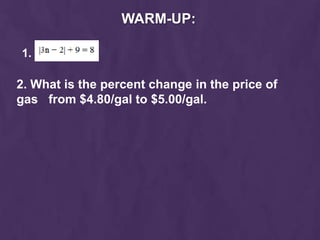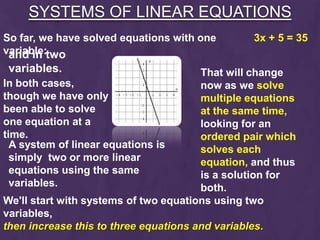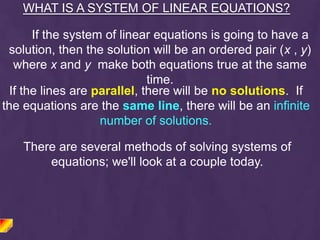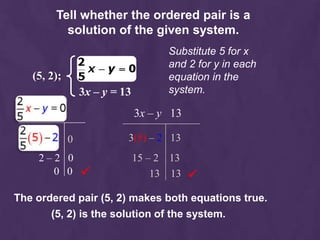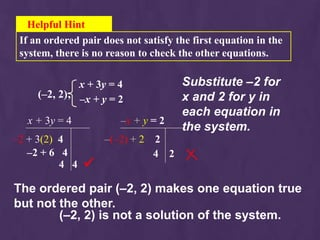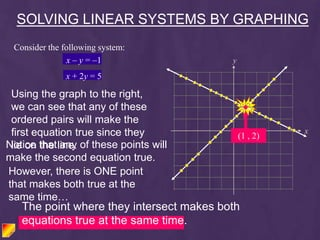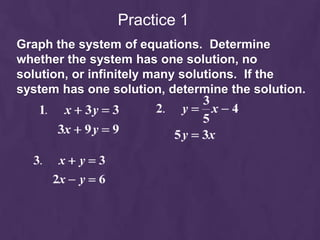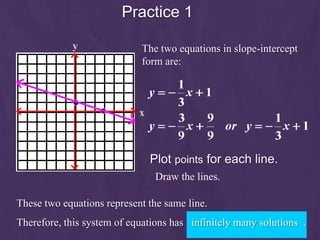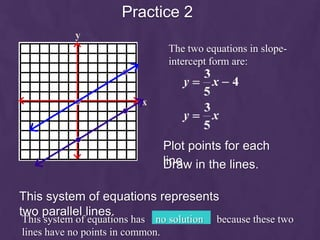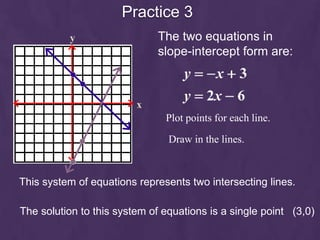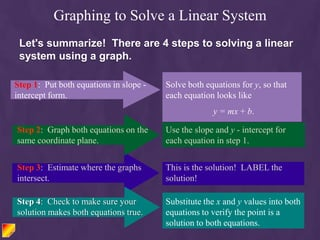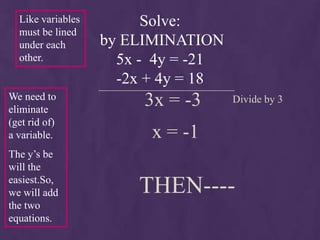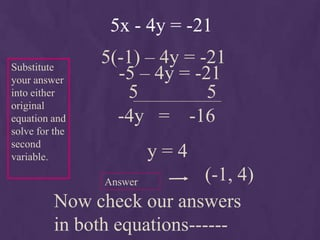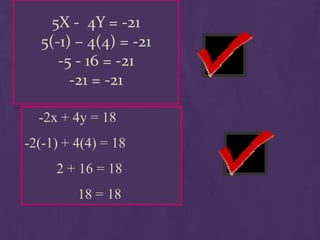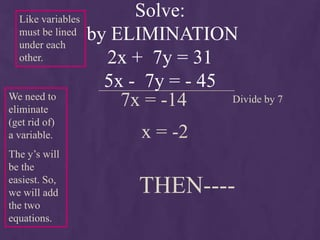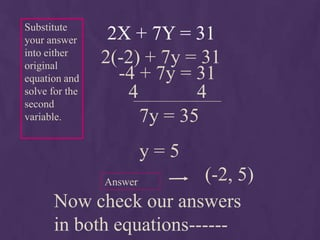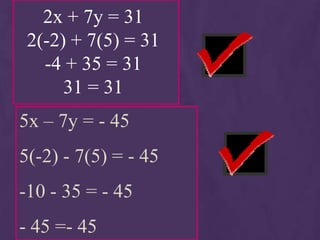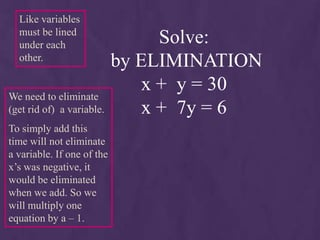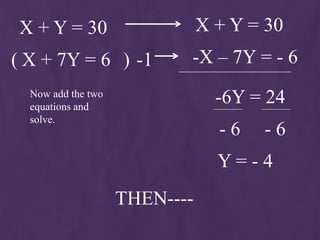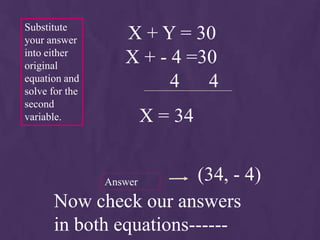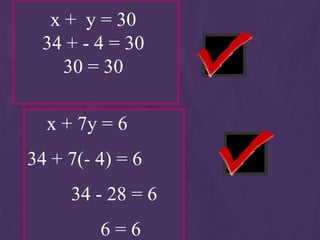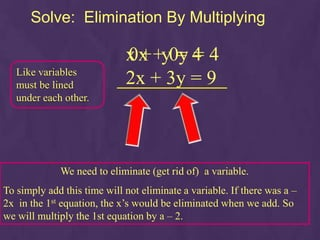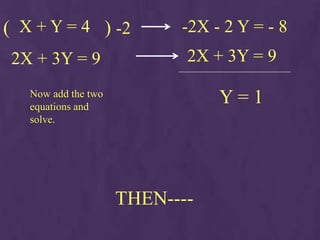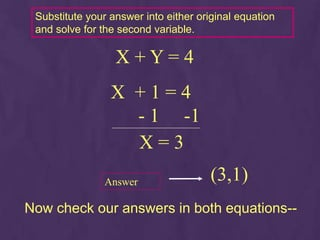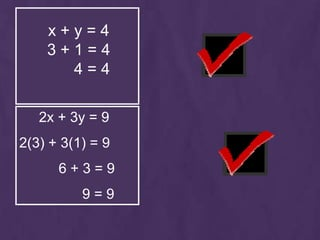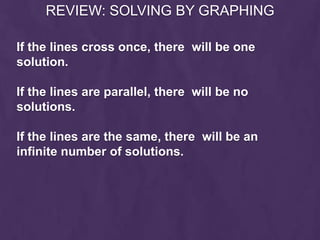1 von 28

### January18

• 1. TODAY: MAKE UP TESTS? **KHAN ACADEMY DUE TOMORROW BEGIN SYSTEMS OF EQUATIONS
• 2. WARM-UP: 1. 2. What is the percent change in the price of gas from \$4.80/gal to \$5.00/gal.
• 3. SYSTEMS OF LINEAR EQUATIONS So far, we have solved equations with one 3x + 5 = 35 variable:two and in variables. That will change In both cases, now as we solve though we have only multiple equations been able to solve at the same time, one equation at a looking for an time. ordered pair which A system of linear equations is solves each simply two or more linear equation, and thus equations using the same is a solution for variables. both. We'll start with systems of two equations using two variables, then increase this to three equations and variables.
• 4. WHAT IS A SYSTEM OF LINEAR EQUATIONS? If the system of linear equations is going to have a solution, then the solution will be an ordered pair (x , y) where x and y make both equations true at the same time. If the lines are parallel, there will be no solutions. If the equations are the same line, there will be an infinite number of solutions. There are several methods of solving systems of equations; we'll look at a couple today.
• 5. Tell whether the ordered pair is a solution of the given system. Substitute 5 for x and 2 for y in each (5, 2); equation in the 3x – y = 13 system. 3x – y 13 0 3(5) – 2 13 2–2 0 15 – 2 13 0 0  13 13  The ordered pair (5, 2) makes both equations true. (5, 2) is the solution of the system.
• 6. Helpful Hint If an ordered pair does not satisfy the first equation in the system, there is no reason to check the other equations. x + 3y = 4 Substitute –2 for (–2, 2); –x + y = 2 x and 2 for y in each equation in x + 3y = 4 –x + y = 2 the system. –2 + 3(2) 4 –(–2) + 2 2 –2 + 6 4 4 2 4 4  The ordered pair (–2, 2) makes one equation true but not the other. (–2, 2) is not a solution of the system.
• 7. SOLVING LINEAR SYSTEMS BY GRAPHING Consider the following system: x – y = –1 y x + 2y = 5 Using the graph to the right, we can see that any of these ordered pairs will make the first equation true since they x (1 , 2) Notice that line. of these points will lie on the any make the second equation true. However, there is ONE point that makes both true at the same time… The point where they intersect makes both equations true at the same time.
• 8. Practice 1 Graph the system of equations. Determine whether the system has one solution, no solution, or infinitely many solutions. If the system has one solution, determine the solution.
• 9. Practice 1 y The two equations in slope-intercept form are: x Plot points for each line. Draw the lines. These two equations represent the same line. Therefore, this system of equations has infinitely many solutions .
• 10. Practice 2 y The two equations in slope- intercept form are: x Plot points for each line. in the lines. Draw This system of equations represents two parallel lines. This system of equations has no solution because these two lines have no points in common.
• 11. Practice 3 y The two equations in slope-intercept form are: x Plot points for each line. Draw in the lines. This system of equations represents two intersecting lines. The solution to this system of equations is a single point (3,0)
• 12. Graphing to Solve a Linear System Let's summarize! There are 4 steps to solving a linear system using a graph. Step 1: Put both equations in slope - Solve both equations for y, so that intercept form. each equation looks like y = mx + b. Step 2: Graph both equations on the Use the slope and y - intercept for same coordinate plane. each equation in step 1. Step 3: Estimate where the graphs This is the solution! LABEL the intersect. solution! Step 4: Check to make sure your Substitute the x and y values into both solution makes both equations true. equations to verify the point is a solution to both equations.
• 13. Like variables Solve: must be lined under each by ELIMINATION other. 5x - 4y = -21 -2x + 4y = 18 We need to eliminate 3x = -3 Divide by 3 (get rid of) a variable. x = -1 The y’s be will the easiest.So, we will add THEN---- the two equations.
• 14. 5x - 4y = -21 5(-1) – 4y = -21 Substitute your answer -5 – 4y = -21 into either 5 5 original equation and -4y = -16 solve for the second variable. y=4 Answer (-1, 4) Now check our answers in both equations------
• 15. 5X - 4Y = -21 5(-1) – 4(4) = -21 -5 - 16 = -21 -21 = -21 -2x + 4y = 18 -2(-1) + 4(4) = 18 2 + 16 = 18 18 = 18
• 16. Like variables Solve: must be lined under each by ELIMINATION other. 2x + 7y = 31 5x - 7y = - 45 We need to eliminate 7x = -14 Divide by 7 (get rid of) a variable. x = -2 The y’s will be the easiest. So, we will add THEN---- the two equations.
• 17. Substitute your answer 2X + 7Y = 31 into either original 2(-2) + 7y = 31 equation and -4 + 7y = 31 solve for the second 4 4 variable. 7y = 35 y=5 Answer (-2, 5) Now check our answers in both equations------
• 18. 2x + 7y = 31 2(-2) + 7(5) = 31 -4 + 35 = 31 31 = 31 5x – 7y = - 45 5(-2) - 7(5) = - 45 -10 - 35 = - 45 - 45 =- 45
• 19. Like variables must be lined under each Solve: other. by ELIMINATION x + y = 30 We need to eliminate (get rid of) a variable. x + 7y = 6 To simply add this time will not eliminate a variable. If one of the x’s was negative, it would be eliminated when we add. So we will multiply one equation by a – 1.
• 20. X + Y = 30 X + Y = 30 ( X + 7Y = 6 ) -1 -X – 7Y = - 6 Now add the two equations and -6Y = 24 solve. -6 -6 Y=-4 THEN----
• 21. Substitute your answer X + Y = 30 into either original X + - 4 =30 equation and solve for the 4 4 second variable. X = 34 Answer (34, - 4) Now check our answers in both equations------
• 22. x + y = 30 34 + - 4 = 30 30 = 30 x + 7y = 6 34 + 7(- 4) = 6 34 - 28 = 6 6=6
• 23. Solve: Elimination By Multiplying x + +y0y 4 4 0x = = Like variables must be lined 2x + 3y = 9 under each other. We need to eliminate (get rid of) a variable. To simply add this time will not eliminate a variable. If there was a – 2x in the 1st equation, the x’s would be eliminated when we add. So we will multiply the 1st equation by a – 2.
• 24. ( X + Y = 4 ) -2 -2X - 2 Y = - 8 2X + 3Y = 9 2X + 3Y = 9 Now add the two equations and Y=1 solve. THEN----
• 25. Substitute your answer into either original equation and solve for the second variable. X+Y=4 X +1=4 - 1 -1 X=3 Answer (3,1) Now check our answers in both equations--
• 26. x+y=4 3+1=4 4=4 2x + 3y = 9 2(3) + 3(1) = 9 6+3=9 9=9
• 27. REVIEW: SOLVING BY GRAPHING If the lines cross once, there will be one solution. If the lines are parallel, there will be no solutions. If the lines are the same, there will be an infinite number of solutions.